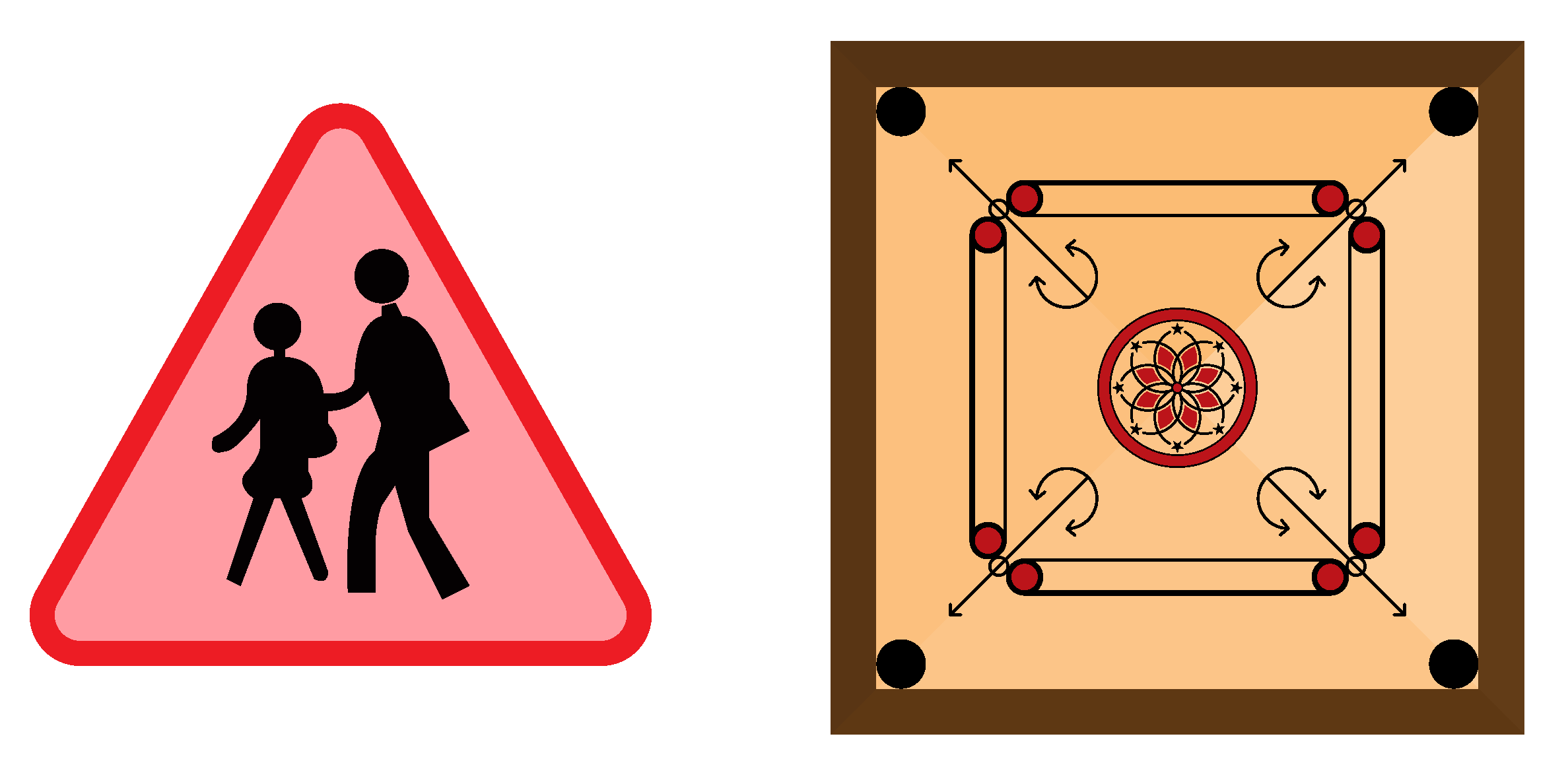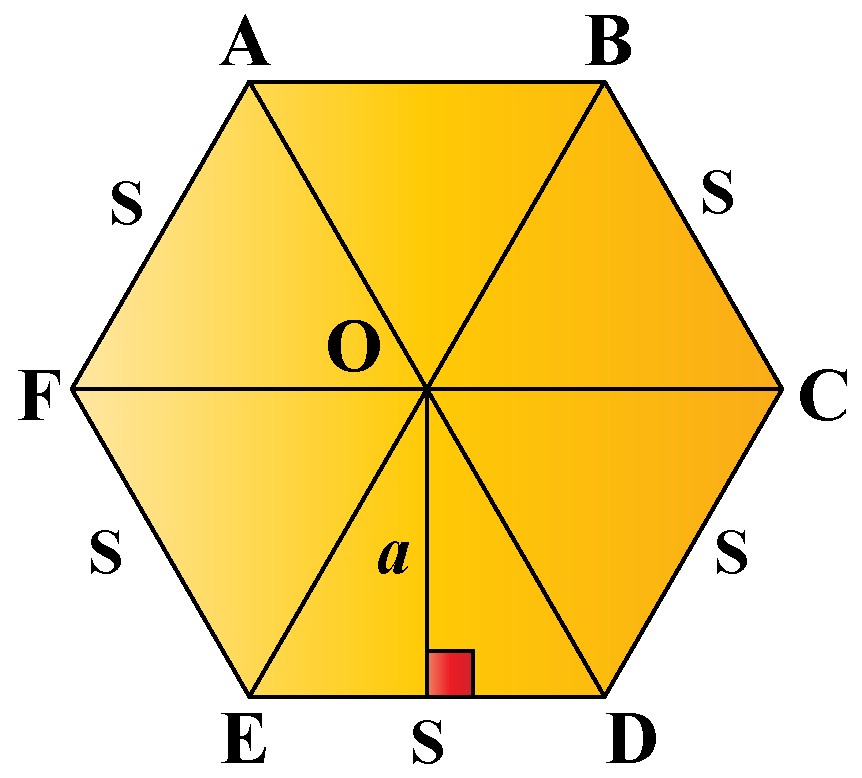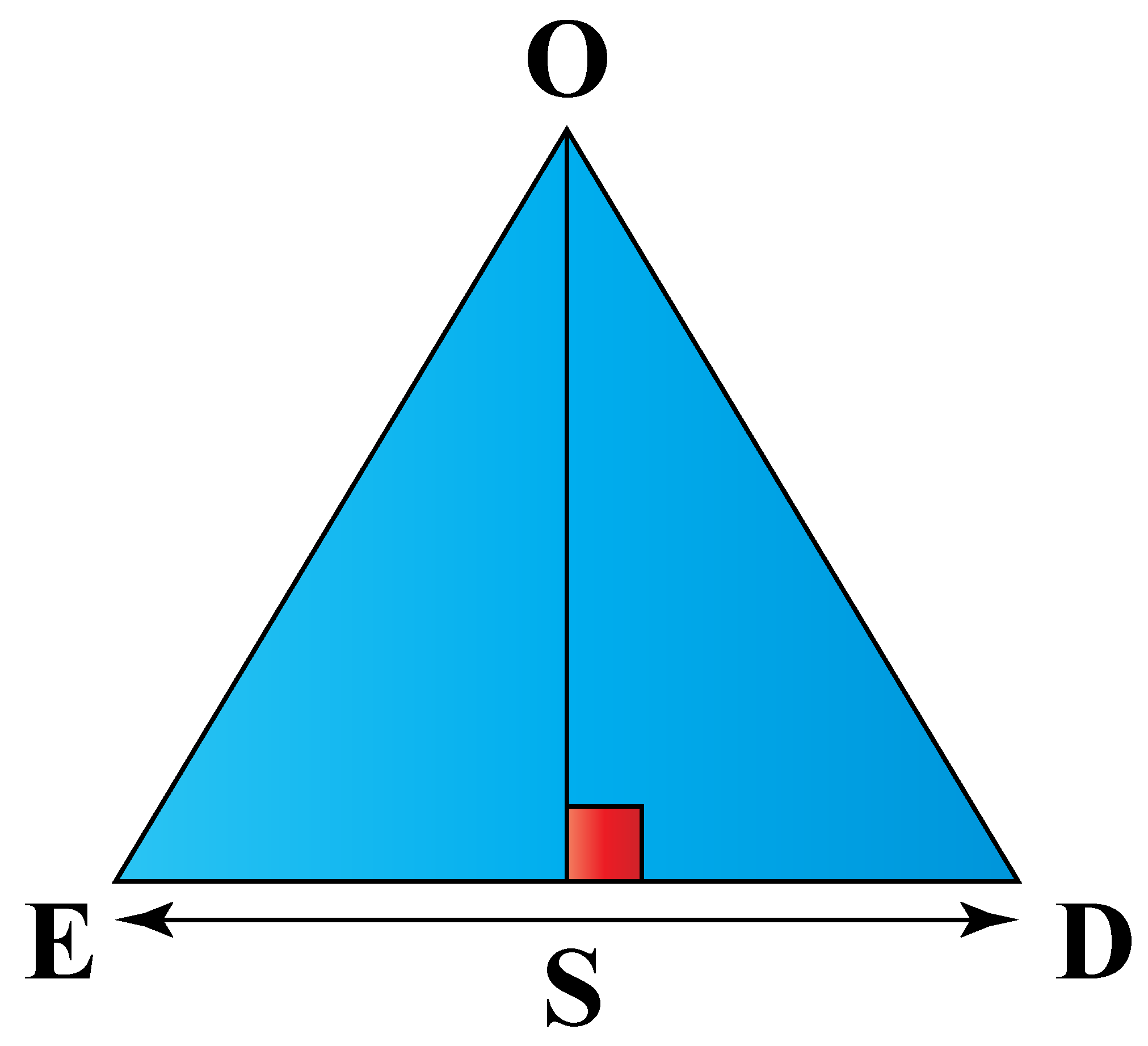# Area of a Regular Polygon

Area of a Regular Polygon
Go back to  'Area'

In this mini-lesson, we will explore about area of a regular polygon by finding about area of a regular polygon using the area of polygon calculator. But before you get your hands on the calculator, it's vital that you know the area of a regular polygon formula and regular polygon definition, as blindly using the calculator will lose its purpose.

Did you know that the first known pentagram to humankind was the pentagram? It is a regular star-shaped polygon that was discovered by Aristophanes.

Over millennia, other polygons were discovered and named based on the number of sides they had. If you look at the simulation below, you too can identify polygons based on the number of sides.

## What is a Regular Polygon?

As per Euclidean geometry, a regular polygon is a polygon that has equal sides and equal angles.

Polygons having sides more than 4 are prefixed with the word "regular".

Regular polygons are convex i.e., all the interior angles measure less than 180º.

Some real-life examples of regular polygons are listed below.Look around you and try to find some regular polygons.

## What is the Formula to Calculate the Area of a Regular Polygon?

The area of a regular polygon formula is given as follows:

 Area of a regular polygon = $$\dfrac{n \times s \times a}{2}$$

where $$n$$ is the number of sides, $$s$$ is the length of one side, and $$a$$ is known as apothem(it is the line from the center of the regular polygon that is perpendicular to one of its sides. It can also be called as the radius of the regular polygon).

The product of the number of sides (n) to the length of one side (s) is the perimeter of the regular polygon. It is given as $$P = n \times s$$.

Hence, the area of a regular polygon in terms of perimeter is given as follows:

 Area of a regular polygon = $$\dfrac{P \times a}{2}$$Tips and Tricks

• Length of apothem for a regular polygon having n sides = $$\dfrac{s}{2 \times \tan(\frac{\pi}{n})}$$.
• If the length of one side is given, it possible to find the area of the regular polygon by finding apothem.

## How to Find Area of a Regular Polygon?

Let's take an example of a regular hexagon.

A regular hexagon can be broken into six triangles, by its diagonals.

Let the length of apothem be "a", length of sides be "s", and center of hexagon is "O".Considering $$\bigtriangleup$$OED we get,Area of $$\bigtriangleup$$OED = $$\dfrac{base \times height}{2}$$

Here base = s and height = a.

Hence,

Area of $$\bigtriangleup$$OED = $$\dfrac{s \times a}{2}$$

There are five other triangles like $$\bigtriangleup$$OED, hence, the area of a regular hexagon can be given by summing each one of them or multiplying the area of one triangle by the number of sides.

Similarly, the area of a regular hexagon can be given by multiplying the area of one triangle by the "n" number of sides as below,

Area of the regular polygon = $$\dfrac{n \times s \times a}{2}$$

To find the area of a regular polygon,

• Step 1: Find apothem using the formula $$\dfrac{s}{2 \times \tan(\frac{\pi}{n})}$$.
• Step 2: Find the area of regular polygon taking the value of apothem obtained in Step 1, and substituting it in the formula $$\dfrac{n \times s \times a}{2}$$.
 Example 1

Ashley bought a square photo frame having the length of each side as $$5$$ inches. Help her find the area the photo frame will occupy?Solution

Ashley bought a square photo frame which is a regular polygon with 4 sides.

To find the area the photo frame will occupy, she will follow the below steps:

• Step 1: Find apothem using the formula $$\dfrac{s}{2 \times \tan(\frac{\pi}{n})}$$.

Here, s = 5 inches and n =4.

Hence, length of apothem = $$\dfrac{5}{2 \times \tan(\frac{\pi}{4})}$$.

The value of angle $$\tan(\frac{\pi}{4})$$ can be obtained from trigonometric ratios.

Hence, length of apothem, a = $$\dfrac{5}{2 \times 1} = 2.5$$ inches.

• Step 2: Find the area of regular polygon taking the value of apothem obtained in Step 1, and substituting it in the formula $$\dfrac{n \times s \times a}{2}$$.

Area of the polygon = $$\dfrac{4 \times 5 \times 2.5}{2} = 25$$ sq. inches.

 $$\therefore$$ Area occupied by square photo frame is $$25$$ sq. inches.
 Example 2

Help Beth find the area of a regular polygon having a perimeter of 35 inches such that the maximum number of sides it has, is less than 7Solution

To find the area of the regular polygon, Beth will use the following steps:

If the perimeter of a regular polygon is given 35 inches, the shape could either have 5 sides or 7 sides.

As per the given question, the maximum number of sides is less than 7, hence the shape of the polygon has 5 sides, the length of each side is 7 inches.

Beth will use the following steps:

• Step 1: Find apothem using the formula $$\dfrac{s}{2 \times \tan(\frac{\pi}{n})}$$.

Here, s = 7 inches and n = 5

Hence, length of apothem = $$\dfrac{7}{2 \times \tan(\frac{\pi}{5})}$$.

Hence, length of apothem, a = $$\dfrac{7}{2 \times 0.726} = 4.82$$ inches.

• Step 2: Find the area of regular polygon taking the value of apothem obtained in Step 1, and substituting it in the formula $$\dfrac{n \times s \times a}{2}$$.

Area of the polygon = $$\dfrac{7 \times 5 \times 4.82}{2} = 84$$ sq. inches.

 $$\therefore$$ Area of a regular polygon is $$84$$ sq. inches.
 Example 3

Stephen has to determine the area of a regular polygon if:

1. The number of sides is doubled
2. The length of the side is halved
3. The number of sides is halved
4. The length of the side is doubledCan you help him determine the answers?

Solution

Stephen knows the area of a regular polygon formula is $$\dfrac{n \times s \times a}{2}$$.

1. If the number of sides is doubled

In this case, $$n = 2n$$

The area of a regular polygon formula now becomes $$\dfrac{(2n) \times s \times a}{2} = n \times s \times a$$.

1. If the length of the side is halved

In this case, s = $$\frac{s}{2}$$

The area of a regular polygon formula now becomes $$\dfrac{n \times \frac{s}{2} \times a}{2} = \dfrac{n \times s \times a}{4}$$.

1. If the number of sides is halved

In this case, $$n = \frac{n}{2}$$

The area of a regular polygon formula now becomes $$\dfrac{\frac{n}{2} \times s \times a}{2} = \dfrac{n \times s \times a}{4}$$.

1. If the length of the side is doubled

In this case, $$s = 2s$$

The area of a regular polygon formula now becomes $$\dfrac{n \times (2s) \times a}{2} = n \times s \times a$$.

 $$\therefore$$ Stephen found answers to all four cases.

## Interactive Questions

Here are a few activities for you to practice.

## Let's Summarize

We hope you enjoyed learning about the area of a regular polygon, area of a regular polygon formula, and regular polygon definition with the simulations, practice questions, and area of a regular polygon calculator.

The mini-lesson targeted the fascinating concept of the area of a regular polygon. The math journey around co-prime numbers starts with what a student already knows, and goes on to creatively crafting a fresh concept in the young minds. Done in a way that not only it is relatable and easy to grasp, but also will stay with them forever. Here lies the magic with Cuemath.

At Cuemath, our team of math experts is dedicated to making learning fun for our favorite readers, the students!

Through an interactive and engaging learning-teaching-learning approach, the teachers explore all angles of a topic.

Be it worksheets, online classes, doubt sessions, or any other form of relation, it’s the logical thinking and smart learning approach that we at Cuemath believe in.

## 1. Are all sides equal in a regular polygon?

Yes, all sides are equal in a regular polygon.

## 2. How do you find the perimeter of a polygon?

The perimeter of a polygon is found by summing the length of all the sides of the polygon.

## 3. What is a regular polygon?

A regular polygon is a polygon that has all sides of the same length and all the angles measuring the same.

More Important Topics
Numbers
Algebra
Geometry
Measurement
Money
Data
Trigonometry
Calculus